# NMath User's Guide

17.8 Hermitian Banded Matrices (.NET, C#, CSharp, VB, Visual Basic, F#)

A Hermitian banded matrix is a Hermitian matrix (Section 17.4) that has all its non-zero entries near the diagonal. Entries farther away from the diagonal than the half bandwidth are defined to be zero. That is, if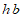is the half bandwidth, then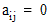wheneveror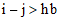. For example, this is a 5 x 5 Hermitian banded matrix with a half bandwidth of 1: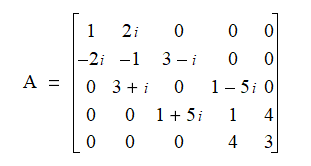According to the strict definition of a Hermitian matrix, the diagonal elements must be real numbers, since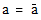only for real numbers), while other elements may be complex. NMath relaxes this requirement and permits complex elements on the diagonal. The provided MakeDiagonalReal() method sets the imaginary parts on the main diagonal to zero, thereby meeting the strict definition of a Hermitian matrix.

NMath provides Hermitian banded matrix classes for single- and double-precision complex numbers. The classnames are and . A symmetric banded matrix is a special case of a Hermitian banded matrix where all the elements are real (Section 17.7).

For efficiency, the lower triangular part of the matrix and zero elements outside the bandwidth are not stored. The storage scheme is the same as for a symmetric banded matrix (Section 17.7).

Top

Top